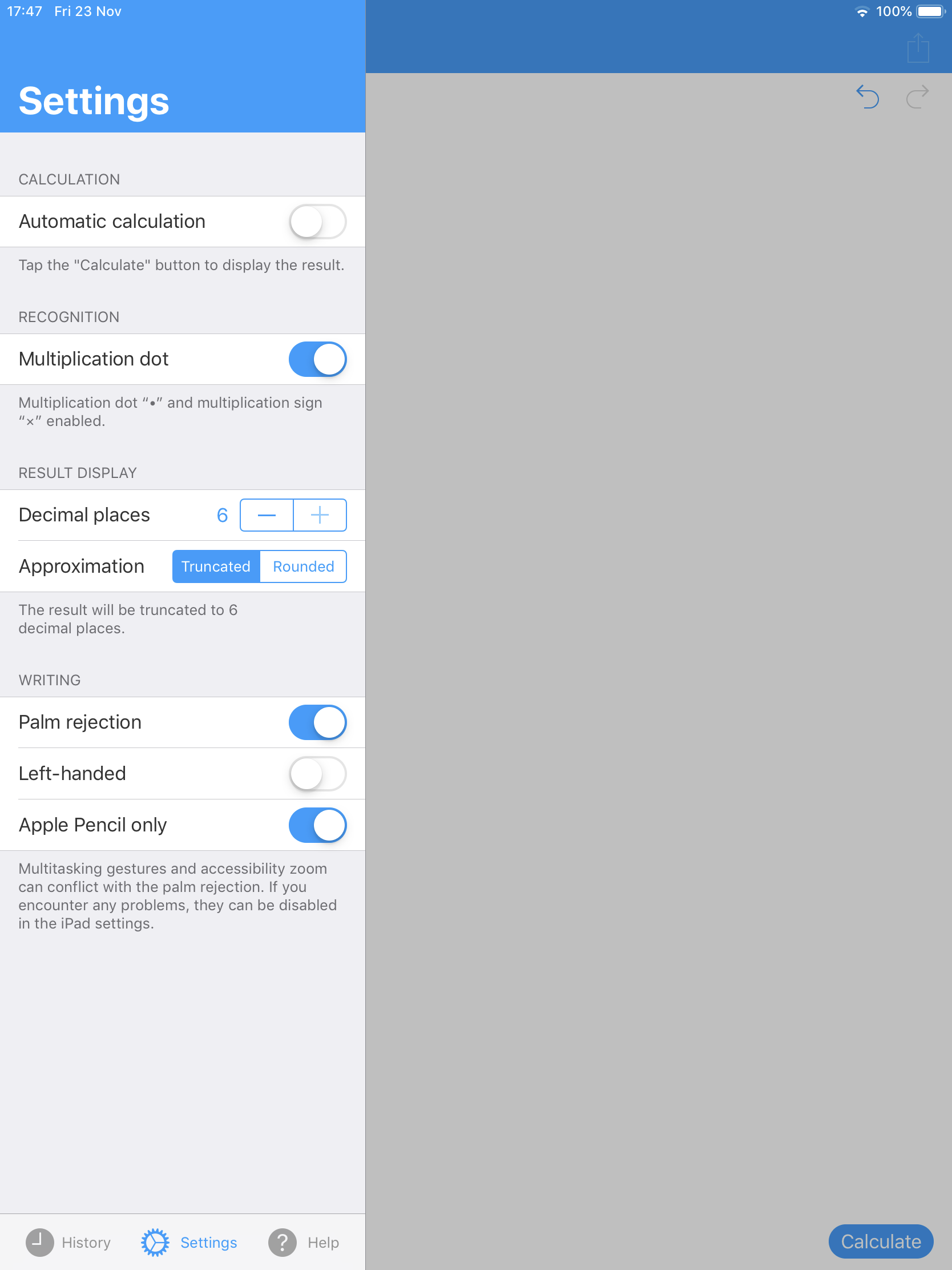# Calculator 2.0 - FAQ

Q) What is MyScript® Calculator 2?

MyScript® Calculator 2 is a tool that performs mathematical operations naturally, using your handwriting. It is easy, simple and intuitive, so just write the mathematical expression on the screen then let MyScript technology perform its magic converting symbols and numbers to digital text and delivering the result in real time. MyScript® Calculator provides the same experience as writing on paper with the advantages of a digital device (editing, solving and export).

Q) What operations does MyScript Calculator 2 support?

MyScript© Calculator supports horizontal calculations, using the following operators and functions:

Basic operations: +, -, x, ÷, +/-, 1/x
Misc. Operations: %, √, x!, |x|
Powers/ Exponentials: ex, xy, x2
Brackets:( )
Trigonometry: cos, sin, tan, cot, cosh, sinh, tanh
Inverse trigonometry: acos (cos -1), asin (sin -1), atan (tan -1)
Logarithms: ln, log, logx
Constants: π, e,φ

You can write one calculation on several lines, and even several calculations on several lines on the same screen. However, columnar or vertical operations are not supported.

Q) Do I need to learn a special way of writing to use MyScript Calculator 2?

No, just write your calculation on the screen, as you would do on paper. No need to learn a new way to write numbers or mathematical symbols, just write naturally.

Q) iOS: Can I use my Apple Pencil?

Yes, make sure the Apple Pencil Only setting is enabledNo, it is no longer available. With the introduction of Calculator 2 the free Calculator 1 version that was available for Android devices has been discontinued. There will be no further updates for Calculator 1 and we are no longer able to provide support for this discontinued app.

Q) Android: Can I use my active pen?

Yes, make sure the Only active pen setting is enabled

Q) What gestures does MyScript Calculator support 2?

Erase: Use scratch-out, strike-through or cross-out to erase any expression or number.
Overwrite: Write on top of a character or symbol to replace it.

Q) Can I write an equation with x and y variables?

MyScript Calculator 2 does not support operations with multiple variables. However, it can be used to solve an equation with one variable. Use the ‘?’ sign as the variable you’re searching for in your mathematical expression, e.g.: 4 x ? = 20

Q) Can I write equations on several lines?

Yes, MyScript Calculator 2 now offers multiline equation recognition.
You can write one calculation on several lines, and even several calculations on several lines on the same screen.Q) Why do I get the following result: -32 = -9? It should be 9!

MyScript Calculator 2 complies with the usual mathematical operators precedence. In that case, the exponent has priority over the subtraction. Hence this operation is interpreted as -(3 x 3) = -9. Put the negative number in brackets to get the expected result: (-3)2 = 9.

Q) What thousand and decimal separators can I use?

MyScript Calculator 2 does not support thousand separators. If you write a comma, it will be interpreted as a decimal separator. The dot vertically centered will be recognized as the interpunct multiplication sign (also called middle dot).

Q) iOS: What Apple devices are supported?

On iOS, MyScript Calculator 2 requires any Apple device with iOS11 and up.

Q) Android: What Android devices are supported?

On Android, MyScript Calculator 2 requires any Android device with Android 5 and up.

Q) How can I convert the result of my calculation?

If you choose to have your calculation solved, the result can either be displayed in decimal form, in fraction or in mixed number.

To choose how the result is to be displayed: Either tap the result then the appropriate display mode or tap the bottom left hand corner button of the app and switch to the appropriate display mode.